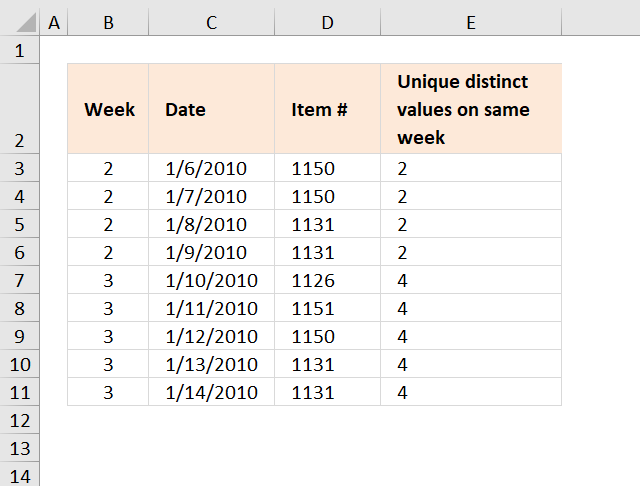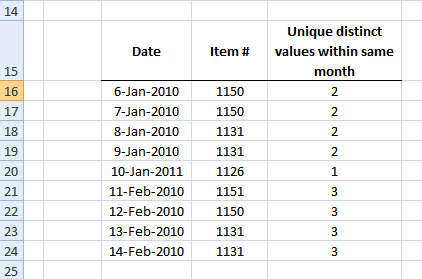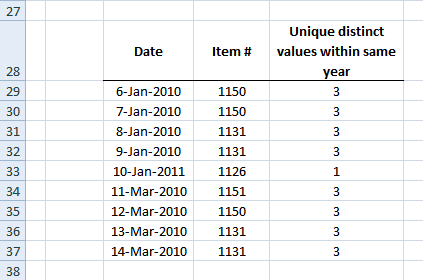Author: Oscar Cronquist Article last updated on December 20, 2018The array formula in cell E3 counts unique distinct items for all dates within the same week. Example, week 2 has two unique distinct items: 1150 and 1131.

Unique distinct values are all values but duplicates are merged into one value.

Formula in B3:

=WEEKNUM(C3) + ENTER

Array formula in E3:

=SUM(--((FREQUENCY(IF(YEAR(C3)&"-"&B3=YEAR(\$C\$3:\$C\$11)&"-"&\$B\$3:\$B\$11, Item, ""), Item))>0))

How to create an array formula

1. Copy (Ctrl + c) and paste (Ctrl + v) array formula into formula bar.
2. Press and hold Ctrl + Shift.
3. Press Enter once.
4. Release all keys.

Copy cell E3 and paste it down as far as needed.

Item is named range pointing to cell range D3:D11.

### Explaining array formula in cell E3

=SUM(--((FREQUENCY(IF(YEAR(C3)&"-"&B3=YEAR(\$C\$3:\$C\$11)&"-"&\$B\$3:\$B\$11, Item, ""), Item))>0))

Step 1 - Filter records in date range

=SUM(--((FREQUENCY(IF(YEAR(C3)&"-"&B3=YEAR(\$C\$3:\$C\$11)&"-"&\$B\$3:\$B\$11, Item, ""), Item))>0))

IF(YEAR(C3)&"-"&B3=YEAR(\$C\$3:\$C\$11)&"-"&\$B\$3:\$B\$11, Item, "")

becomes

IF("2010-2"={"2010-2";"2010-2";"2010-2";"2010-2";"2011-3";"2010-3";"2010-3";"2010-3";"2010-3"}, Item, "")

becomes

IF({TRUE;TRUE;TRUE;TRUE;FALSE;FALSE;FALSE;FALSE;FALSE}, {1150; 1150; 1131; 1131; 1126; 1151; 1150; 1131; 1131}, "")

and returns

{1150;1150;1131;1131;"";"";"";"";""}

Step 2 - Calculate frequency

=SUM(--((FREQUENCY(IF(YEAR(C3)&"-"&B3=YEAR(\$C\$3:\$C\$11)&"-"&\$B\$3:\$B\$11, Item, ""), Item))>0))

FREQUENCY(IF(YEAR(C3)&"-"&B3=YEAR(\$C\$3:\$C\$11)&"-"&\$B\$3:\$B\$11, Item, ""), Item))

becomes

FREQUENCY({1150;1150;1131;1131;"";"";"";"";""}, {1150; 1150; 1131; 1131; 1126; 1151; 1150; 1131; 1131}))

and returns

{2;0;2;0;0;0;0;0;0;0}

Step 3 - Count and sum values larger than 0 (zero)

=SUM(--((FREQUENCY(IF(YEAR(C3)&"-"&B3=YEAR(\$C\$3:\$C\$11)&"-"&\$B\$3:\$B\$11, Item, ""), Item))>0))

becomes

=SUM(--(({2;0;2;0;0;0;0;0;0;0})>0))

becomes

=SUM(--({TRUE; FALSE; TRUE; FALSE; FALSE; FALSE; FALSE; FALSE; FALSE; FALSE}))

becomes

=SUM({1;0;1;0;0;0;0;0;0;0})

and returns 2 in cell E3.

### Get Excel *.xlsx file

Count-unique-occurences-within-same-week-month-year.xlsx

### Count unique distinct values within same month### Array formula in E16:

=SUM(--(FREQUENCY(IF(YEAR(C16)&"-"&MONTH(C16)=YEAR(\$C\$16:\$C\$24)&"-"&MONTH(\$C\$16:\$C\$24), \$D\$16:\$D\$24, ""), (\$D\$16:\$D\$24))>0))

### How to create an array formula

1. Copy (Ctrl + c) and paste (Ctrl + v) array formula into formula bar.
2. Press and hold Ctrl + Shift.
3. Press Enter once.
4. Release all keys.

Copy cell E16 and paste it down as far as needed.

### Count unique distinct values within same year### Array formula in E29:

=SUM(--(FREQUENCY(IF(YEAR(C29)=YEAR(\$C\$29:\$C\$37), \$D\$29:\$D\$37, ""), (\$D\$29:\$D\$37))>0))

### How to create an array formula

1. Copy (Ctrl + c) and paste (Ctrl + v) array formula into formula bar.
2. Press and hold Ctrl + Shift.
3. Press Enter once.
4. Release all keys.

Copy cell E29 and paste it down as far as needed.

### Get excel sample file

Count-unique-occurences-within-same-week-month-year.xls